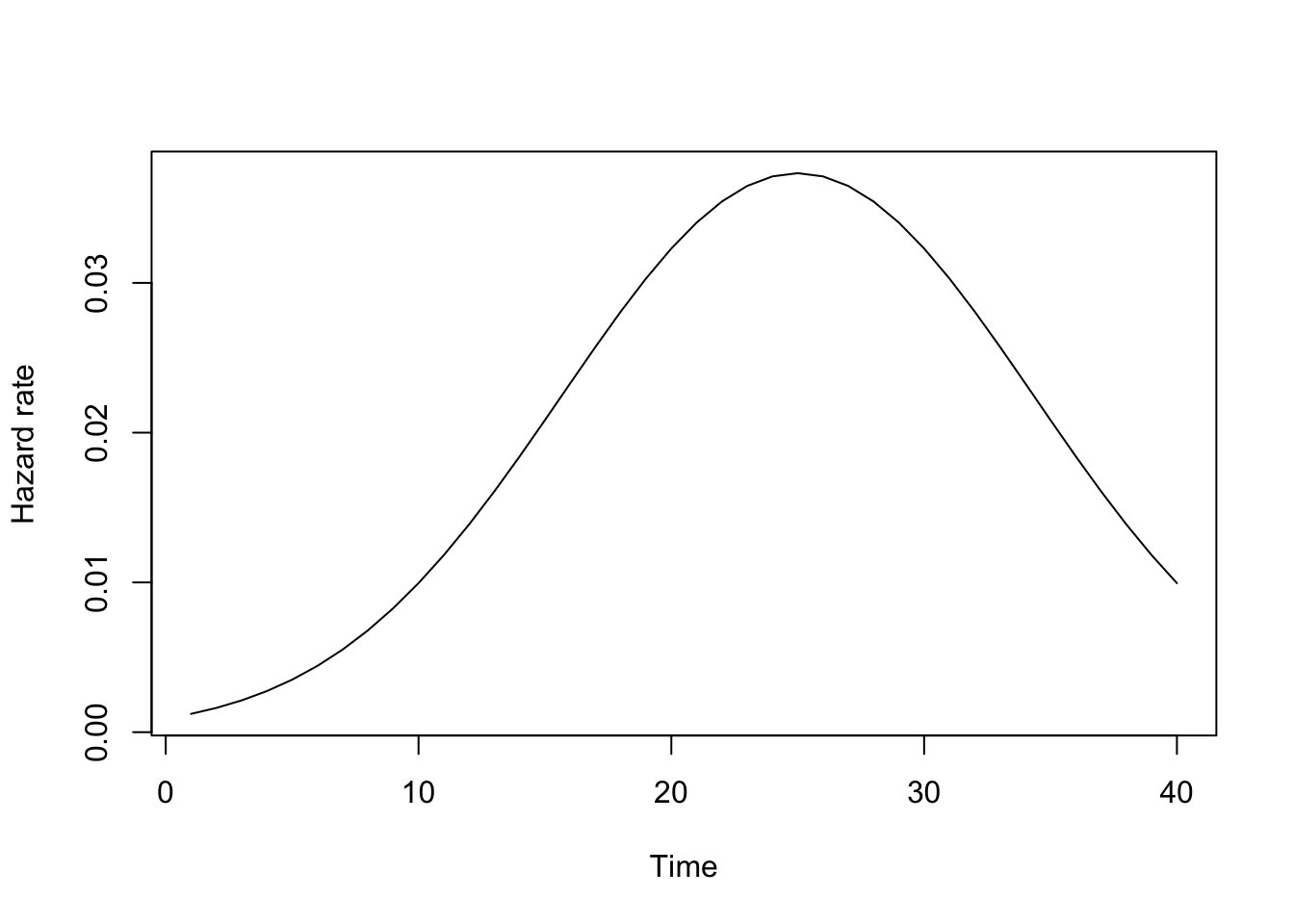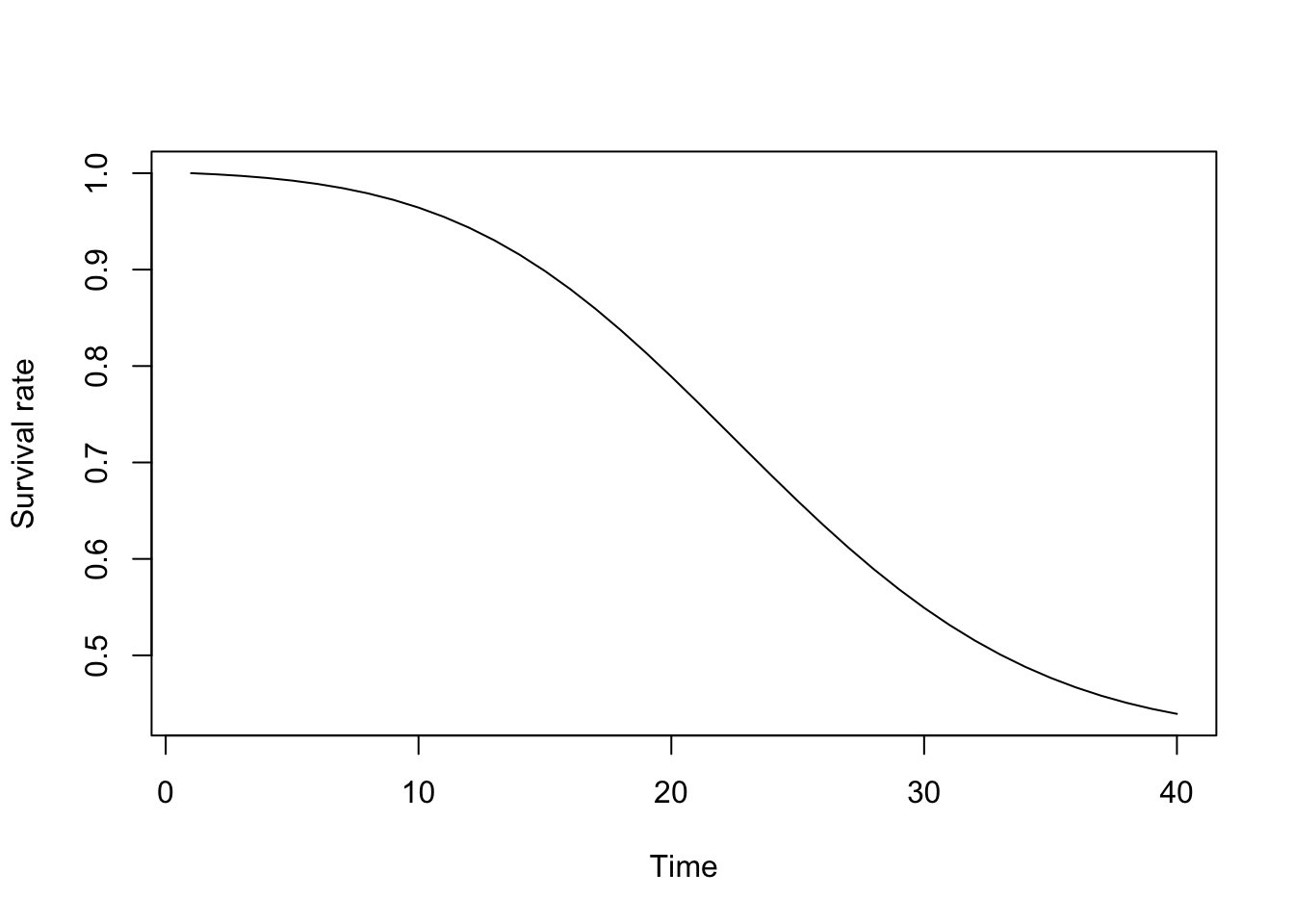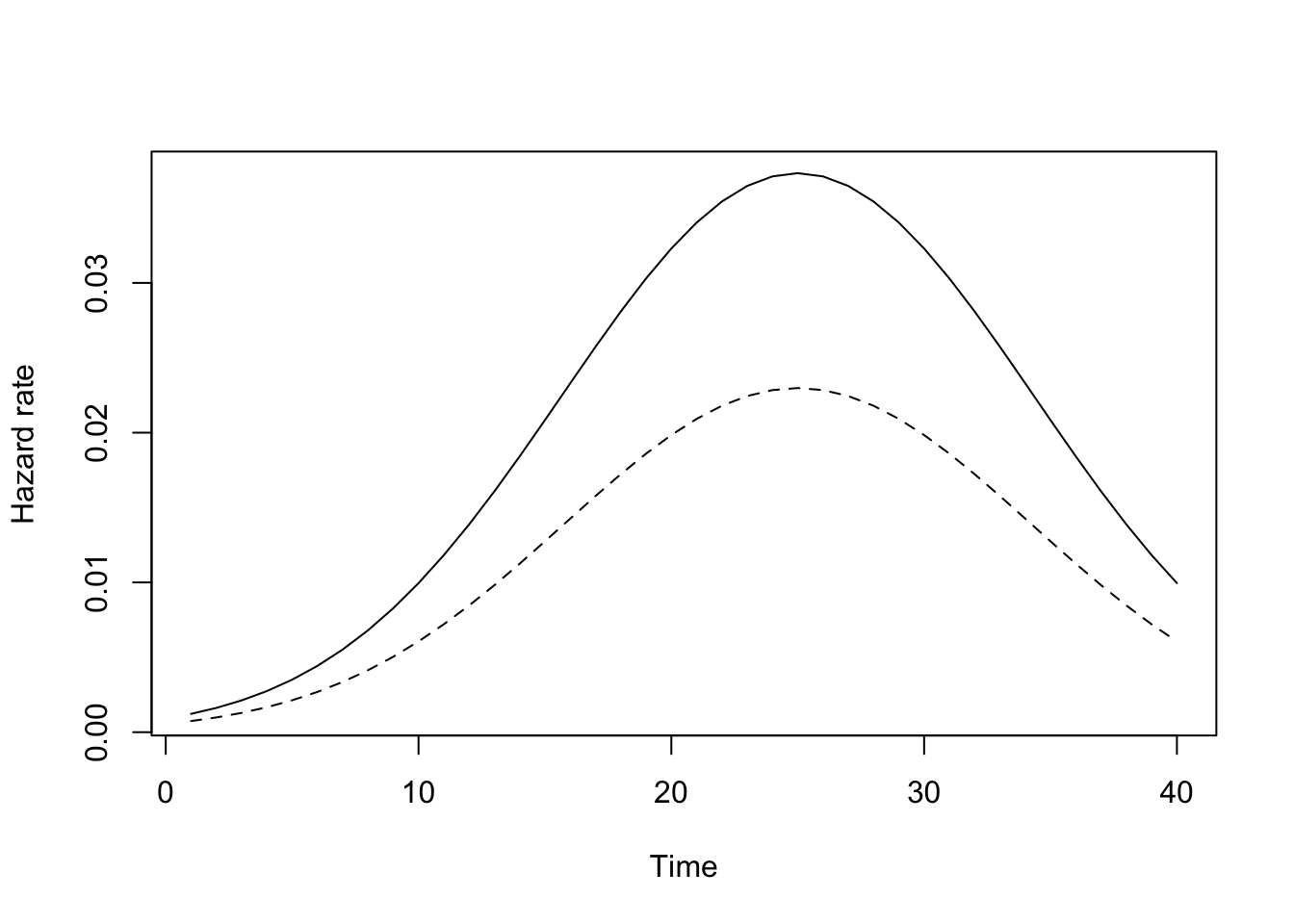Note: This document is uploaded on Ryota Mugiyama’s website.

## Definition of hazard and discrete-time logit model

Hazard rate is defined as follows.

$r(t) = \lim_{\Delta t \rightarrow 0} \frac{\Pr(t \leq T < t + \Delta t | T \geq t)}{\Delta t}$ In discrete-time framework, this is reformalized as follows.

$r(t) = \Pr(T = t | T \geq t)$

We can estimate this hazard rate by using binary logit model. If the baseline-hazard follows the bell-shaped curve (that is, quadatic function), the hazard rate model is represented as the following logit model. $r(t) = \frac{\exp(\beta_0 + \beta_1 t + \beta_2 t^2)}{1 + \exp(\beta_0 + \beta_1 t + \beta_2 t^2)}$ Or,

$\log \frac{r(t)}{1 - r(t)} = \beta_0 + \beta_1 t + \beta_2 t^2$

## How to calculate hazard and survival rate

For example, consider we obtain the following estimates. $\log \frac{r(t)}{1 - r(t)} = -7 -0.3t -0.006t^2$

t <- 1:40
c1 <- 0.3*t - 0.006*t^2 - 7 # estimated slopes and intercept.
r1 <- exp(c1) / (1 + exp(c1)) # calculate predicted hazard rate.
plot(t, r1, type = "l", ylab = "Hazard rate", xlab = "Time")We can calculate the survival rate by using the previous logit model. The survival rate is defined as follows.

$S(t) = \Pr(T > t) = \prod^t_{t = 1} (1 - r(t))$ After we estimate predicted hazard rate, we can calculate the predicted survival rate. Following table is the image of calculating survival rate by the predicted hazard rate.

Time Survival rate Hazard rate
1 $$S(1) = 1$$ $$\hat{h}(1)$$
2 $$\hat{S}(2) = \hat{S}(1) \times (1 - \hat{h}(1))$$ $$\hat{h}(2)$$
3 $$\hat{S}(3) = \hat{S}(2) \times (1 - \hat{h}(2))$$ $$\hat{h}(3)$$
4 $$\hat{S}(4) = \hat{S}(3) \times (1 - \hat{h}(3))$$ $$\hat{h}(4)$$
s1 <- (rep(1, 40))
for (i in 2:40) {
s1[i] <- s1[i-1] * (1 - r1[i-1]) # calculate survival rate.
}
plot(t, s1, type = "l", ylab = "Survival rate", xlab = "Time")## Two heterogenious population

Consider there are two heterogenious population; One is the group with high hazard, the other is with low hazard. $\mathrm{Group} \ A: \log \frac{r(t)}{1 - r(t)} = -7 -0.3t -0.006t^2$ $\mathrm{Group} \ B: \log \frac{r(t)}{1 - r(t)} = -7.5 -0.3t -0.006t^2$

c2 <- c1 - 0.5 # lower hazard group (the difference is intercept only).
r2 <- exp(c2) / (1 + exp(c2))
s2 <- (rep(1, 40))
for (i in 2:40) {
s2[i] <- s2[i-1] * (1 - r2[i-1])
}

plot(t, r1, type = "l", ylab = "Hazard rate", xlab = "Time")
lines(t, r2, type = "l", lty = 2)The survival rates are as follows.

plot(t, s1, type = "l", ylab = "Survival rate", xlab = "Time")
lines(t, s2, type = "l", lty = 2)